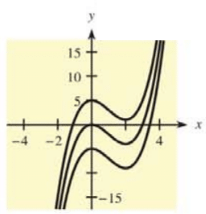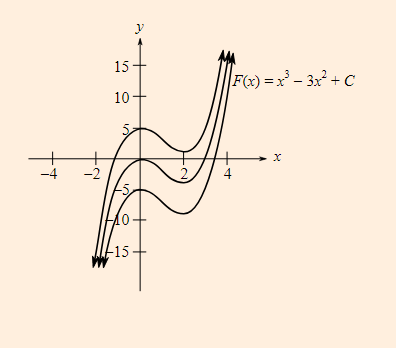Chapter 12.1, Problem 39E### Mathematical Applications for the ...

11th Edition
Ronald J. Harshbarger + 1 other
ISBN: 9781305108042

#### Solutions

Chapter
Section### Mathematical Applications for the ...

11th Edition
Ronald J. Harshbarger + 1 other
ISBN: 9781305108042
Textbook Problem

# In each of Problems 37-40, a family of functions is given and graphs of some members of the family are shown. Write the indefinite integral that gives the family. F ( x ) = x 3 − 3 x 2 + CTo determine

To calculate: The indefinite integral that gives the family of the curves F(x)=x33x2+C.Explanation

Given Information:

The provided equation is,

F(x)=x33x2+C

And, the family of curves is,

Formula used:

According to the power rule of derivatives,

ddx(xn)=nxn1

Calculation:

Consider the provided function,

F(x)=x33x2+C

Now, to obtain the indefinite integral that gives the above family of curves, first differentiate both sides with respect to x as,

ddx(F(x))=ddx</

### Still sussing out bartleby?

Check out a sample textbook solution.

See a sample solution

#### The Solution to Your Study Problems

Bartleby provides explanations to thousands of textbook problems written by our experts, many with advanced degrees!

Get Started

#### The difference between population variance and sample variance.

Statistics for The Behavioral Sciences (MindTap Course List)

#### True or False: converges.

Study Guide for Stewart's Multivariable Calculus, 8th

#### Self Check Multiply:3+-252--9.

College Algebra (MindTap Course List)